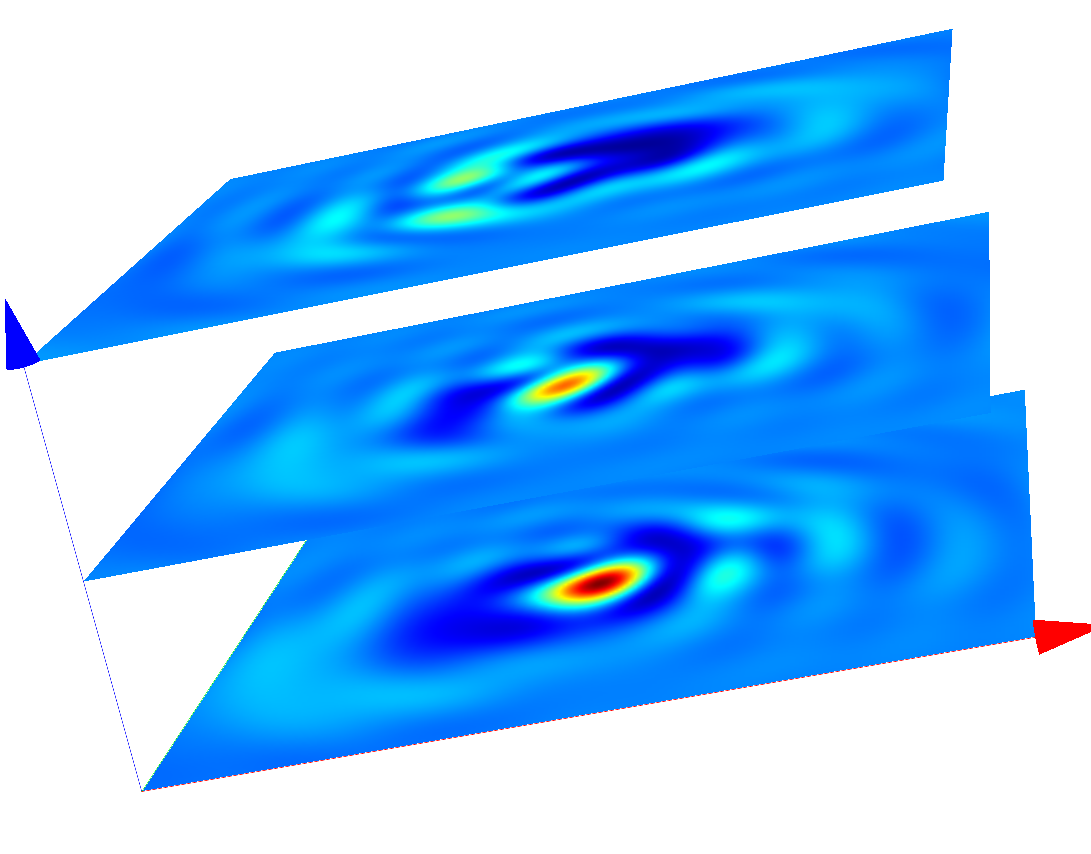# Cross¶

This example computes light propagation of incident plane waves (at oblique angle of incidence) through an isolated cross:

Note

As the geometry of this setup exhibits two mirror symmetries the computational domain is reduced to a quarter domain (at higher numerical efficiency).

In a first post process, the Fourier transform for the upper half space is computed (output file `transmitted_fourier_transform.jcm`). In a subsequent post-process `OpticalImaging` this Fourier transform is mapped to `image_fourier_transform.jcm` modelling the light transfer through the optical system (as defined in the post-process `OpticalImaging`). You can use a Cartesian export post-process to compute the so formed coherent image. The following figure shows the image in different-slices (image planes displaced along-direction), for-polarized illumination.

The following figure shows the image in different-slices (image planes displaced along-direction), for-polarized illumination.Coherent images of the cross after passing the optical system (s-polarized incoming plane wave with oblique incidence)Coherent images of the cross after passing the optical system (p-polarized incoming plane wave with oblique incidence)